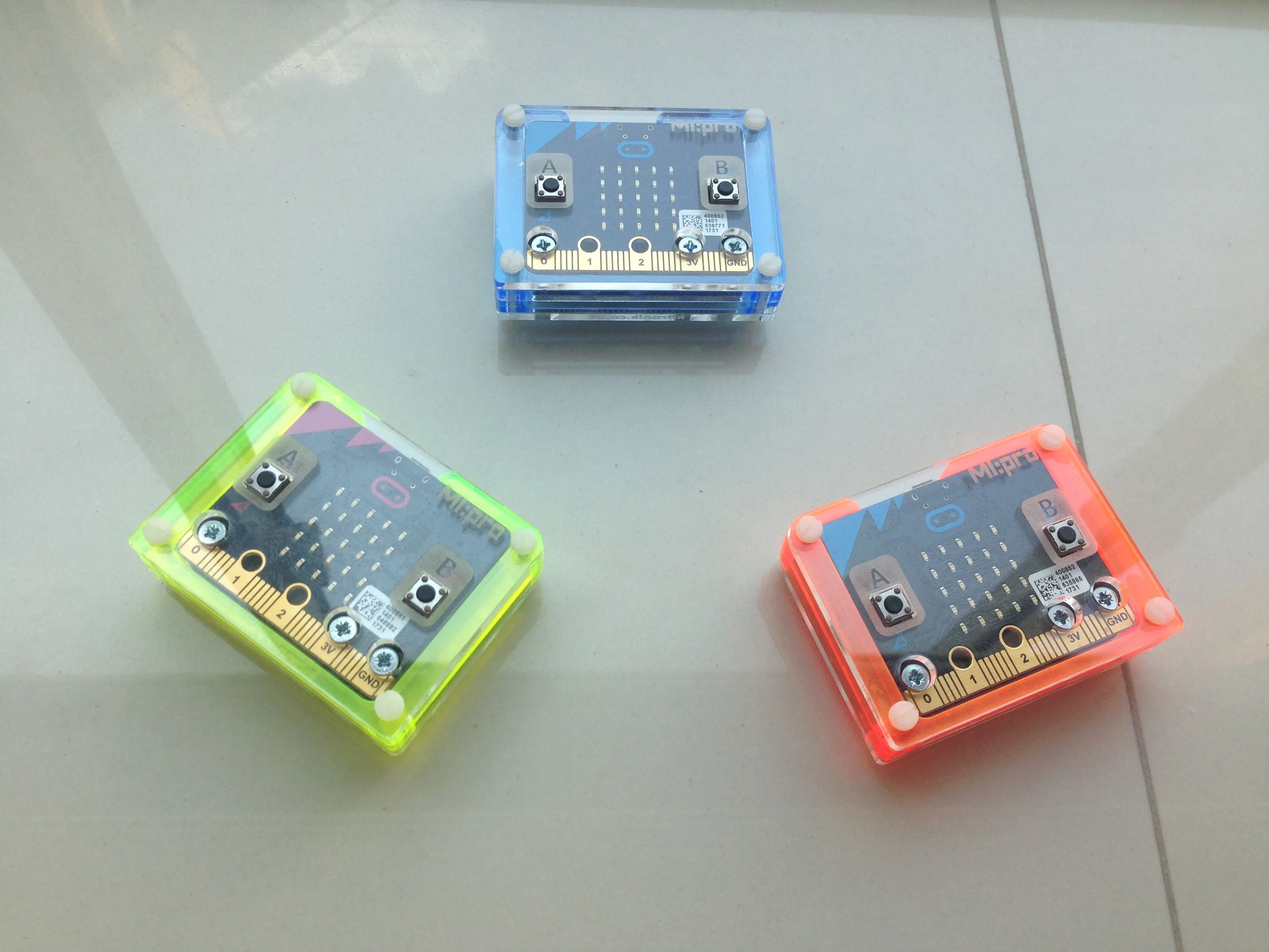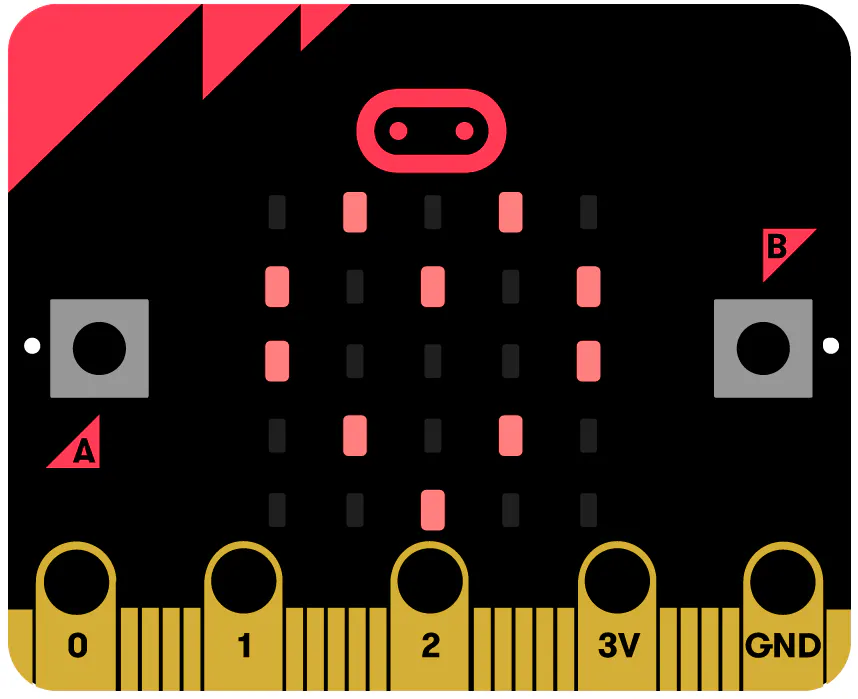Published

# Competitive Math Training Thru Micro:bits (Client Version)

A Master Micro:bit (teacher) issues math equations to Client Micro:bits (students). Students then compete to be first w/ the correct answer.

IntermediateFull instructions provided1 hour740## Things used in this project

### Hardware componentsBBC micro:bit board
×1
 Kitronik MI:Power Board
×1
 Kitronik MI:pro Protector Case
×1

## Code

### A Microbit-Based Competitive Math Training Game (Client Version)

JavaScript
The Client program of a Microbit-based competitive math training game.
```let PressButtB = 0
let PressButtA = 0
let B1 = 0
let WinSw = 0
let MsgEqOld = ""
let A1 = 0
let ShowCorrSw = 0
let Wins = 0
let MsgEq = ""
let PressOthButt = 0
let PressOthButtB = 0
let GotCorrect = 0
let X = 0
let MyDeviceNo = 0
let Y = 0
if (name == "PLUS") {
Y = value % 100
X = value - Y
X = X / 100
MsgEq = "" + X + "+" + Y
if (MsgEq != MsgEqOld) {
MsgEqOld = MsgEq
ShowCorrSw = 0
GotCorrect = 0
X = 0
Y = 0
A1 = 0
B1 = 0
PressButtA = 0
PressButtB = 0
PressOthButt = 0
PressOthButtB = 0
WinSw = 0
for (let i = 0; i < 3; i++) {
basic.clearScreen()
}
PressOthButt = 1
PressOthButtB = 1
basic.showString(MsgEqOld)
basic.pause(500)
basic.clearScreen()
}
} else {
if (name == "CID") {
if (value == MyDeviceNo) {
if (GotCorrect == 0) {
GotCorrect = 1
if (ShowCorrSw == 0) {
ShowCorrSw = 1
}
if (WinSw == 0) {
WinSw = 1
Wins += 1
if (Wins == 5) {
ShowCorrSw = 2
Wins = 0
}
}
}
}
} else {
if (name == "RES") {
Wins = 0
WinSw = 0
}
}
}
})
input.onButtonPressed(Button.A, () => {
if (GotCorrect == 0) {
for (let i = 0; i < 2; i++) {
basic.clearScreen()
}
PressOthButtB = 1
if (PressButtA == 0) {
basic.clearScreen()
A1 = 0
basic.showNumber(A1)
basic.pause(500)
PressButtA = 1
PressOthButt = 0
} else {
if (PressOthButt == 1) {
basic.clearScreen()
basic.showNumber(A1)
PressOthButt = 0
basic.pause(500)
} else {
A1 = A1 + 1
if (A1 > 9) {
A1 = 0
}
basic.clearScreen()
basic.showNumber(A1)
basic.pause(500)
}
}
}
})
input.onGesture(Gesture.Shake, () => {
if (GotCorrect == 0) {
basic.clearScreen()
PressOthButt = 1
PressOthButtB = 1
basic.showString(MsgEqOld)
basic.pause(500)
basic.clearScreen()
}
})
input.onButtonPressed(Button.B, () => {
if (GotCorrect == 0) {
basic.clearScreen()
PressOthButt = 1
if (PressButtB == 0) {
basic.clearScreen()
B1 = 0
basic.showNumber(B1)
basic.pause(500)
PressButtB = 1
PressOthButtB = 0
} else {
if (PressOthButtB == 1) {
basic.clearScreen()
basic.showNumber(B1)
PressOthButtB = 0
basic.pause(500)
} else {
B1 += 1
if (B1 > 9) {
B1 = 0
}
basic.clearScreen()
basic.showNumber(B1)
basic.pause(500)
}
}
}
})
input.onButtonPressed(Button.AB, () => {
if (GotCorrect == 0) {
PressOthButt = 1
PressOthButtB = 1
basic.clearScreen()
}
})
MyDeviceNo = control.deviceSerialNumber()
MsgEq = ""
MsgEqOld = ""
GotCorrect = 0
X = 0
Y = 0
A1 = 0
B1 = 0
PressButtA = 0
PressButtB = 0
PressOthButt = 0
PressOthButtB = 0
Wins = 0
WinSw = 0
basic.forever(() => {
}
if (ShowCorrSw != 0) {
for (let i = 0; i < 2; i++) {
basic.clearScreen()
basic.showIcon(IconNames.Yes)
basic.pause(500)
if (ShowCorrSw == 2) {
basic.clearScreen()
basic.showLeds(`
# . . . #
# . . . #
# . # . #
# . # . #
. # . # .
`)
basic.pause(500)
}
}
music.beginMelody(music.builtInMelody(Melodies.PowerUp), MelodyOptions.Once)
}
})
```

## Credits

### Philip Cua

4 projects • 0 followers
Thanks to Marcus Cua.